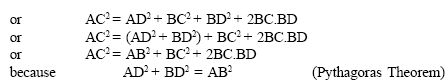Name: ___________________Date:___________________

 Email us to get an instant 20% discount on highly effective K-12 Math & English kwizNET Programs!

### High School Mathematics - 28.14 Pythagorean Theorem - Examples

 Theorem: In a right angled triangle, the square on the hypotenuse is equal to the sum of the squares of the other two sides. If the length of the hypotnuse is c and the lengths of other two sides are b and a then according to the pythogorean theorem: c2 = a2 + b2Converse: In a triangle if the square of one side is equal to the squares on the sums of the other two sides, then the angle opposite to the first side is a right angle. To prove: AB2 + BC2 = AC2Example: Prove that the sum of the squares of the sides of a rhombus is equal to the sum of the squares of the diagonals.Directions: Solve the following problems. Also write at least 10 examples of your own.
 Q 1: A ladder is placed in such a way such that its distance from the wall to the foot of the ladder is 5 cm, its top reaches a height of 12m from the ground. Find the length of the ladder.13m12m15m Q 2: The sides of a triangle are 7cm, 24cm, 25cm, check if this is a right angled triangle.yesno Q 3: The sides of a triangle are 50cm, 80cm, 100cm, check if this is a right angled triangle.yesno Q 4: A person goes 10 m due east and then 30m due north. Find his distance from the starting point.10m12m15m Q 5: Triangle PQR is equilateral. QR = 30 units, B is the midpoint of QA. QA is perpendicular to PR. What is the length of PB? 24 cm23 cm19.84 cm Q 6: A ladder 20 m long reaches a height of 16m above the ground. Find the distance from the foot of the ladder to the wall.17 m13 m12 m Question 7: This question is available to subscribers only! Question 8: This question is available to subscribers only!